# DAV Class 3 Maths Chapter 7 Worksheet 5 Solutions

The DAV Class 3 Maths Solutions and DAV Class 3 Maths Chapter 7 Worksheet 5 Solutions of Money offer comprehensive answers to textbook questions.

## DAV Class 3 Maths Ch 7 WS 5 Solutions

Question 1.
Add the following amounts of money.

(a)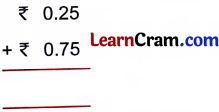Solution:
₹ 1.00

(b)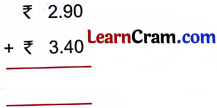Solution:
₹ 6.30(c)Solution:
₹ 33.88

(d)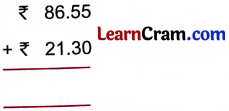Solution:
₹ 107.85

(e)Solution:
₹ 90.00

(f)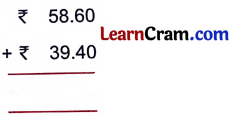Solution:
₹ 98.00Question 2.
Arrange the and add the following amounts of money.

(a) ₹ 47.00, ₹ 78.00 and ₹ 35.00
Solution: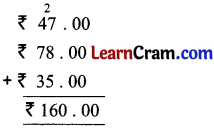(b) ₹ 93.25 and ₹ 64.65
Solution:(c) ₹ 42.05, ₹ 76.75 and ₹ 14.35
Solution: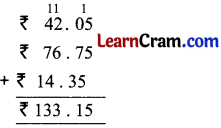(d) ₹ 80.08, ₹ 80.80 and ₹ 88.00
Solution:(e) ₹ 0.97, ₹ 0.09 and ₹ 1.09
Solution:(f) ₹ 143.83, ₹ 134.28 and ₹ 348.23
Solution: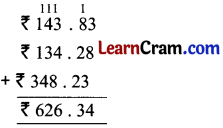### DAV Class 3 Maths Chapter 7 Worksheet 5 Notes